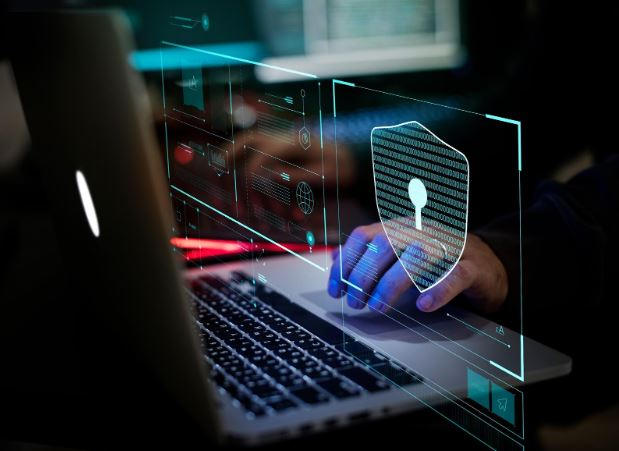# Prime Numbers

by on August 13, 2020

in Security

## Internet Cryptography and the role of Prime numbers

Prime numbers have continuously attracted human attention. It is quite alluring that the entire internet cryptography is based on this mystery.  The American Science Fiction, Contact (1997) is inspired by the book called ‘Contact’ written by Carl Sagan that claims  Aliens tried to speak to/with humans through ‘Prime Numbers’ as signals.

There are loads of fascinating facts concerning prime numbers that make them ‘a mathematical mystery.’ In this blog, we will explore more about Prime Numbers and their significance in Cyber Security and Encryption. We will additionally discover how Cyber Security works using Prime Numbers.

## What is a Prime Number?

Prime numbers are a special set of integers that cannot be divided into multiples of other numbers except ‘1’ and the number itself. A prime number can also be defined as a natural number that has only two factors or divisors. For example, 3 is a prime number that can be divided only by 1 and 3.

Did you notice why 0 and 1 were NOT listed under prime numbers?

• 1 can be divided only by itself and therefore, it has a single factor. Thus, 1 is NOT a prime number.
• 0 can be divided by any number hence, it has several factors. Thus, 0 is NOT a prime number.

Now, try to guess the answer to the following question:

Which is the ONLY even prime number?

The answer is 2, and that is the ONLY even prime number.

## Prime Numbers in Cyber Security

We were introduced to the concept of prime numbers in school but never did we imagine that these numbers are so uniquely interesting!

Prime numbers protect us from cybercrime through the RSA encryption system. You must be wondering how such a basic concept can be used to encrypt data. Let us find out!

How are Prime numbers used for cybersecurity?

Before we proceed further and discuss prime number encryption, let us first understand the meaning of  Cyber Security and encryption.

Cyber Security involves protecting data, such as intellectual property, financial data, personal information, or other types of data, across networks that are at a very high risk of unauthorized access or exposure. Encryption is the process of concealing information by altering it such that it appears as random data. Encryption is essential for security on the Internet.The secrecy with prime numbers

‘For a computer, multiplying two prime numbers, each even 100 digits long, isn’t that difficult; however, factorising the result back into its components is notoriously difficult, even for supercomputers.’ – The RSA encryption system uses this advantage of prime numbers to encrypt data. It uses large prime numbers to form the private and public keys as “the bigger the prime number, the more difficult and time-consuming it is to prime-factor the public key.”

## How does Prime Number Encryption Work?

Anyone can use the public key to encrypt a message, but only someone with knowledge of prime numbers can decode the message.

This method can be better understood by an example.

Assume that C is a very large number and is a product of two prime numbers P and Q. To protect any sensitive data, the data needs to be encrypted. While encrypting, for instance, a customer’s debit card details, the number C is used to generate the “public” key. This key, as its name defines, is available to the public, and it can be seized and read by anyone in the network. Banks are known to use public keys that are 617 digits long to secure customers’ private transactions.

A public key secures private information by locking it in a container whose handles are tightly clasped with a several hundred-digit combination-lock. The container itself can be accessed by anyone, but the content inside cannot be accessed. A thief may secretly steal the container; however, without possessing the “private” key or the combination, the thief cannot unlock it. This private key is only possessed by the sender and receiver of the content — the bank and the respective customer who is the proprietor of the debit card.The private key consists of a subset of two prime numbers P and Q, that are multiplied to produce the public key C. Any thief without the knowledge of those prime numbers has to factorise the public key C, which could take the thief hundreds of years if the numbers are hundreds of digits long, as there are a lot of huge prime numbers.

The largest known prime number is 282,589,933 − 1 (as of January 2020), a number which has 24,862,048 digits when written in base 10. Now, just imagine how difficult it would be to  decode something like that!

## Importance of Prime Numbers in Encryption

Encryption involves a “public key” consisting of the product of two large primes used to encrypt a message, and a “secret key” consisting of those two primes used to decrypt the message. You can make the public key public, and everyone can use it to encrypt messages to you, but only you know the prime factors and can decrypt the messages.

To summarise, I believe that the ‘ mystery of Prime numbers makes the internet a safer place’. Calculations also reveal that prime numbers become rarer as numbers grow larger. It is very difficult to follow a pattern as you count through them; they are more like lottery tickets!

Thank you for spending your time here. I hope it was a good read. Do comment below and let me know what you think of these mysterious numbers!seeksocial August 14, 2020 at 13:55

Hello
Good day!